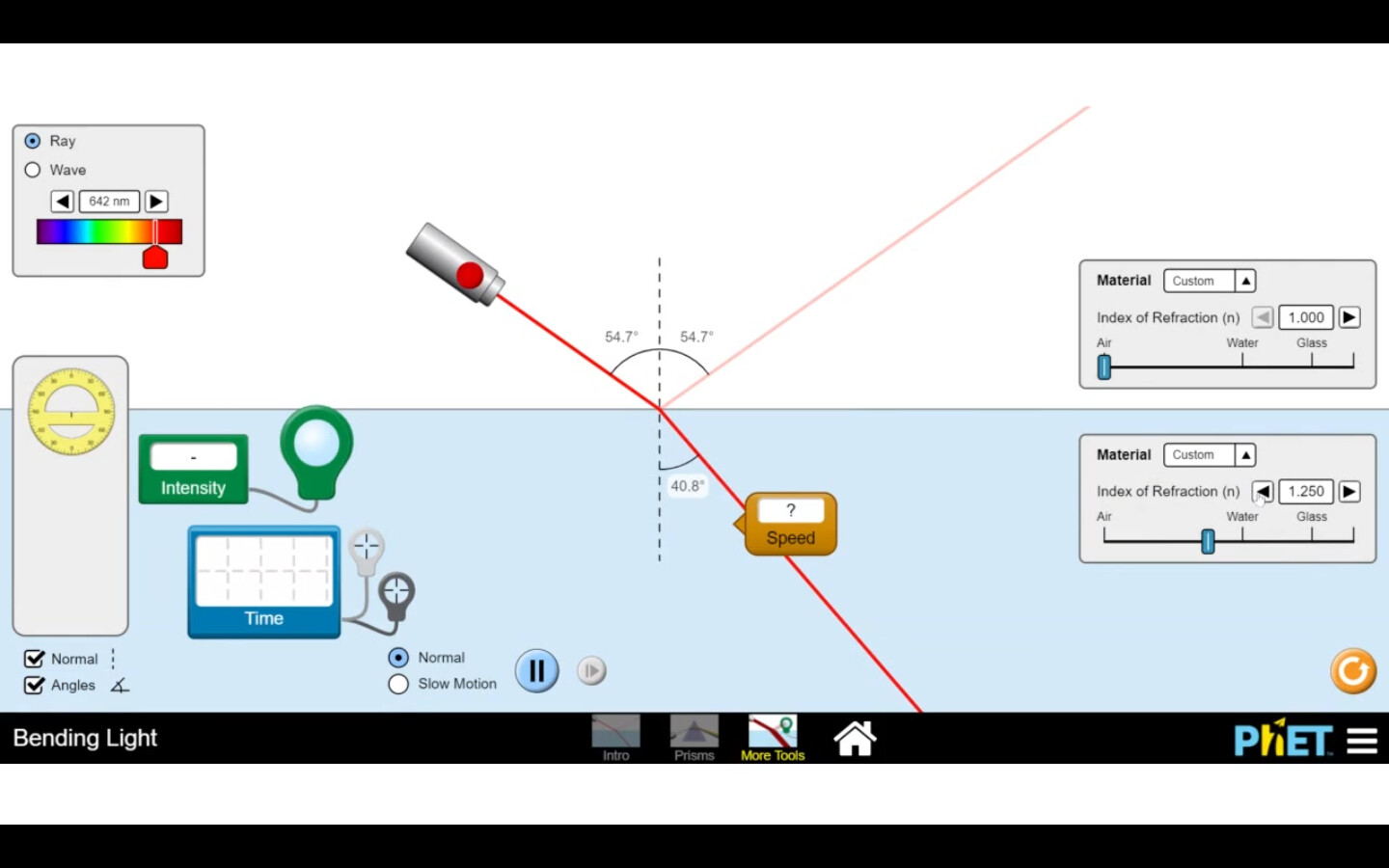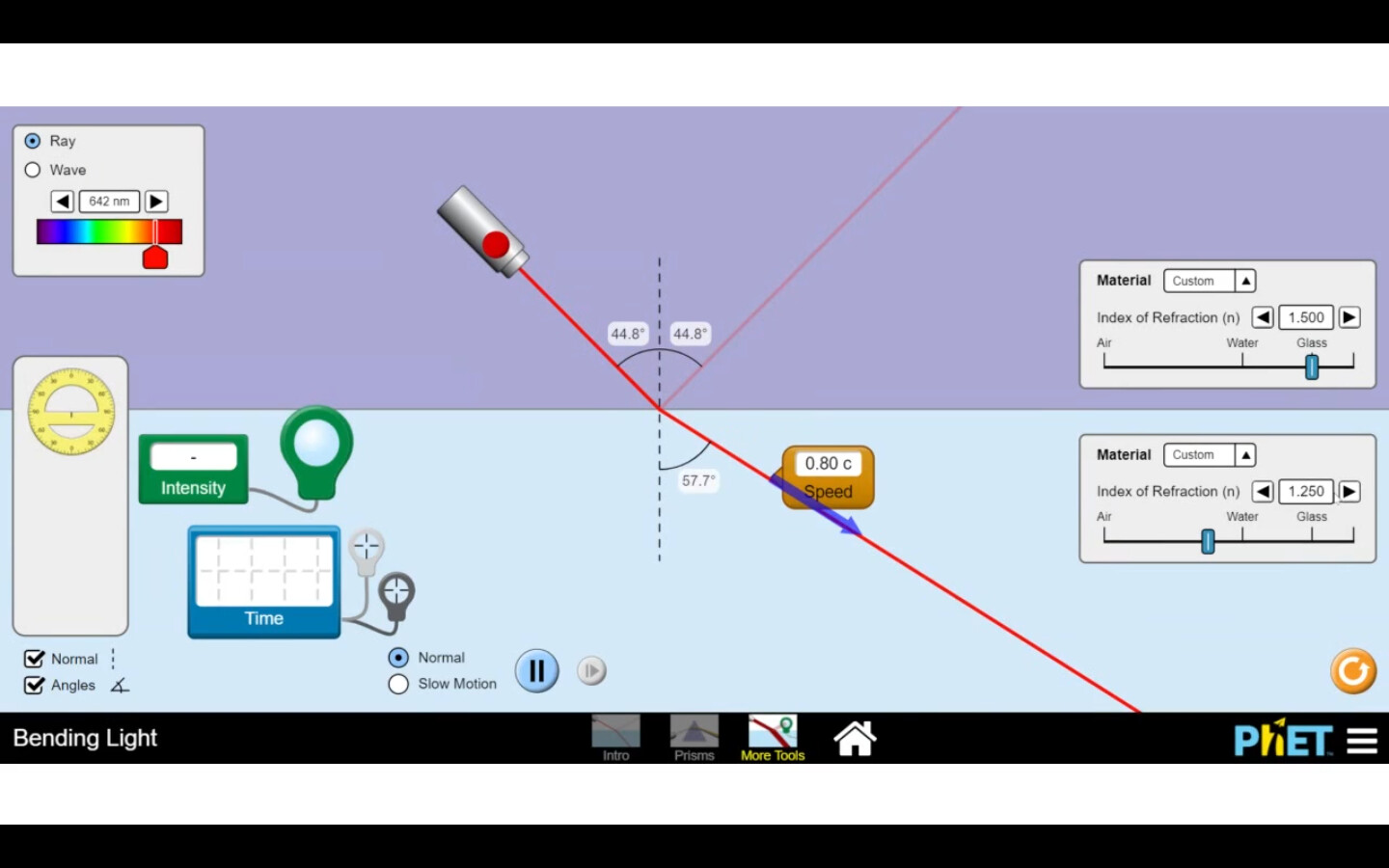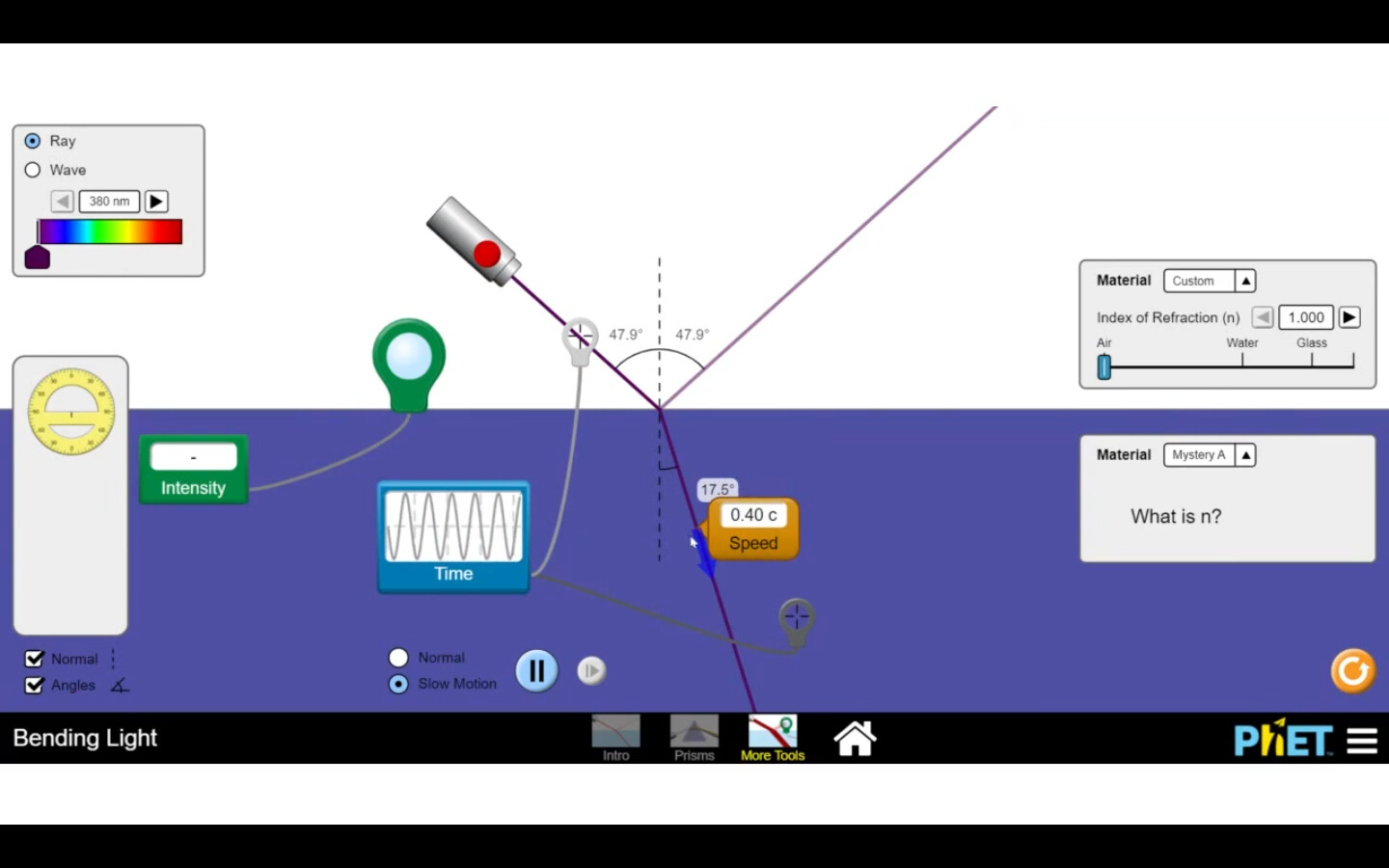# Bending Light - (Part 3) [06 July 2021]

## Bending of Light - Part III

Here is the attempt to explore the properties of light and related phenomena and concepts using the PhET simulation on the same.

Here is the link to the previous discussions

## Observations

• We observed that the speed of light in medium = c /RI of medium
• On changing the wavelength of light, we could see that the refractive index of the medium in the simulation also varied. (On increasing the wavelength of light, the angle of refraction decreased.)
• Observations could be summarized as follows: Less the wavelength, more the RI, less the speed, more the bending (lesser the angle of refraction)
• We predicted the RI of mystery medium B based on exploration, and then empirically verified the same by varying the RI and measuring the light speed using the probe provided in the simulation.

## Exploration prompts

• What is the relationship between the refractive index and the speed of light in the medium?• Does the speed of light on travelling from air to new medium varies with the change in the angle of incidence?

• Suppose the speed of light travelling from air to a medium with a refractive index of 1.25 is 0.80 c. Now, if replace air medium (i.e. medium 1) with a different medium having a RI as 1.5. Will, that change the speed of light in medium 2? Can you predict the speed of light now in medium 1 when its RI is 1.5?• Why does the speed of light change by changing the wavelength of the light?

• Why the angle of refraction changed on changing the wavelength of light?

• Why does the RI of the medium varied on changing the wavelength of light, keeping the other parameters the same?

• How can we predict the RI of mystery medium A in which the speed of light is 0.4c?• Can the wavelength of light vary on passing through different mediums?

Do share your thoughts, suggestions, questions and feedback!

## Acknowledgements

### Discussion

Collaborators in video resource creation: Deepak Arora, Swarnava Mitra, Ravi Sinha

### Credits

1. PhET Interactive Simulations